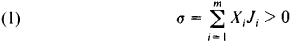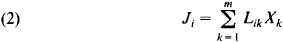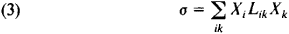# Entropy Production

The following article is from The Great Soviet Encyclopedia (1979). It might be outdated or ideologically biased.

## Entropy Production

the entropy arising in a physical system per unit time as a result of nonequilibrium processes occurring in the system. The entropy production per unit volume is called the local entropy production.

If thermodynamic forces Xi—such as temperature gradients, concentration gradients of components, gradients of the components’ chemical potentials, gradients of mass velocity, and, in heterogeneous systems, the finite differences of thermodynamic parameters—produce in a system the conjugate fluxes Ji—for example, fluxes of heat, matter, and momentum—then the local entropy production σ in such a nonequilibrium system iswhere m is the number of driving thermodynamic forces. The total entropy production is equal to the integral of σ over the system’s volume. If the thermodynamic fluxes and forces are constant in space, then the total entropy production differs from the local production only by a factor equal to the volume of the system. The relation between the fluxes Ji and the thermodynamic forces xi producing the fluxes is given by the linear equationswhere the Lik are the kinetic coefficients (seeONSAGER THEOREM). Consequently, the entropy production isthat is, the entropy production is a quadratic form in the thermodynamic forces.

Entropy production is nonzero and positive for irreversible processes; indeed, the criterion for irreversibility is σ ≠ 0. According to the Prigogine theorem, the entropy production is minimum in stationary states. The specific expression for the kinetic coefficients entering into the entropy production is determined in terms of the interaction potentials of the particles by the methods of nonequilibrium statistical thermodynamics.

D. N. ZUBAREV

References in periodicals archive ?
In this paper, we generate the layer adaptive meshes to suppress the oscillations, via an entropy production operator which is positive automatically whenever the solution is unphysical, which effectively corresponds to insufficient resolution.
In modified theories, an additional entropy production term appeared in Clausius relation that corresponds to the nonequilibrium behavior of thermodynamics while no extra term appears in GB gravity, Lovelock gravity, and braneworld gravity [20-24].
Their topics include fluctuating hydrodynamics and fluctuation-dissipation theorem in equilibrium systems, non-equilibrium thermodynamics for evaporation and condensation, non-equilibrium thermodynamics of interfaces between aqueous solutions and crystals, minimizing entropy production with optimal control theory, mesoscopic non-equilibrium thermodynamics in biology, and the dynamics of complex fluid-fluid interfaces.
The net effect is seen here; that is, entropy production increases only in the centerline of the channel while it decreases at the walls.
Aoki, "Entropy flow and entropy production in the human body in basal conditions," Journal of Theoretical Biology, vol.
The Maximum Entropy Production Principle (MEPP) has been proposed to deal with general theoretical issues of thermodynamics and statistical physics.
The numerical entropy production (NEP) has been implemented successfully as smoothness indicator (error indicator) in adaptive finite volume methods used to solve compressible fluid problems [1-4].
With the choice [j.sub.s] = [j.sub.e]/T, and using (2) and (4), the entropy production is found to be
According to the thermodynamics, the change of entropy should consist of the entropy production and the entropy flow induced by water flow, thermal conduction, and thermal convection.
A recent edition of the Philosophical Transaction of the Royal Society B (May 2010) dedicated itself to the principle of Maximum Entropy Production (MEP), which states that thermodynamic processes far from equilibrium (e.g.

Site: Follow: Share:
Open / Close